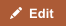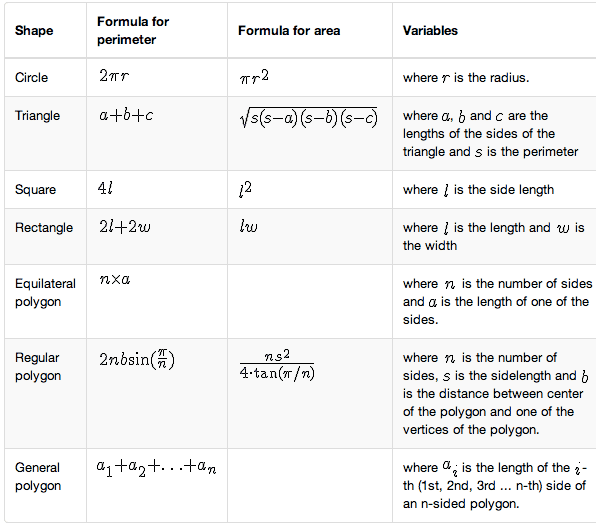In geometry, area is the 2-dimensional space or region occupied by a closed figure, while perimeter is the distance around a closed figure i.e. the length of the boundary. For example, the area can be used to calculate the size of the carpet to cover the whole floor of a room. Perimeter can be used to calculate the length of fence required to surround a yard or garden. Two shapes may have the same perimeter, but different areas or may have the same area, but different perimeters.

## Comparison chart

Area versus Perimeter comparison chartAreaPerimeter
Definition The space or region occupied by a closed figure. The distance around a closed figure.
Measurement Square unit.(sq) Measures two dimensions e.g. 24 in² or 24 inches squared Linear unit Measures one dimension Eg 24 in. or 24 inches
Usage For example to carpet the whole room For example to put a fence around the garden
Square s², where s is the length of one side of the square. 4s, where s is the length of one side of the square.
Rectangle lw, where l and w are the length and width of the rectangle. 2l + 2w, , where l and w are the length and width of the rectangle.
Triangle Sq. root (s*(s-a)(s-b)(s-c)), where s is half the perimeter, a, b and c are the lengths of the sides. OR ½*ab*sin(C), where a and b are any two sides, and C is the angle between them. OR ½*bh, where b is the base and h is height a+b+c, where a,b and c are the lengths of the sides of the triangle.
Rhombus Product of diagonals / 2 4 * l
Trapezium (a+b)/2 Sum of all sides
Parallelogram Length(l) * Height(h) 2 * (length(l) + Breadth(b))
Circle πr², where r is the radius of the circle. 2πr, where r is the radius

## Measurement and Units

Area represents a two-dimensional region; so the unit for area is "square units". e.g. 24 inches squared or 20 centimeters squared. This is written as 20 cm2.

We use linear units when we measure perimeter. Linear units measure one dimension, length.

## Formulas to calculate area and perimeterCheat sheet of mathematical formulas to calculate area and perimeter for a variety of geometric shapes, including circle, square, triangle, rectangle, equilateral polygons, regular polygons and general polygons.

## Irregular Objects

An irregular shape has sides of different length. Calculating area on these shapes involves breaking the shape down into common shapes like squares, rectangles, triangles and circles. This is because these shapes all have set formulas for calculating their area. Being able to see the shapes within shapes is the key to calculating the area of irregular shapes. After finding the area of each shape add them to get the total area. In the case of perimeter of irregular object just measure the length of each side and add them up.